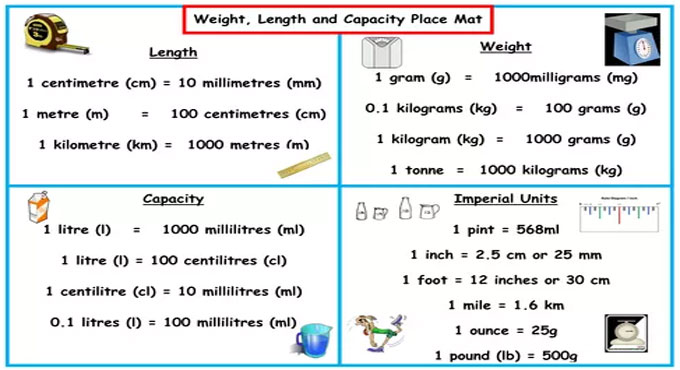# Units of measurement used in surveying & construction worksUnit of measurement is also known as the International System of Units, or SI. Units of measure in the metric system. The units of length or linear size are derived from the metre. They comprise of the kilometre (km) that is 1000 meters, the centimetre (cm), and the millimetre (mm) which is 1/1000th of a meter.

A unit of measurement refers to a specific magnitude of a quantity, described and approved by convention or by law, that is used as a standard for measurement of the same kind of quantity.

Any other quantity of that type can be stated as a multiple of the unit of measurement. As for instance, a length is a physical quantity.

Standard unit of measurement :-

Type - U.S. Unit - Metric Unit
Length - Inches, Feet, Yards, Miles - Millimeters, Centimeters, Meters, Kilometers
Weight - Ounces, Pounds - Grams, Kilograms
Time - Seconds, Minutes, Hours - Seconds, Minutes, Hours
Volume - Ounces, Gallons - Milliliters, Liters

Given below, the details of measurements which are utilized in surveying and construction works :-

For construction work: feet, inches, fractions of inches (m, mm)
For most surveys: feet, tenths, hundredths, thousandths (m, mm)

For National Geodetic Survey (NGS) control surveys: meters, 0.1, 0.01, 0.001 m

The following equivalents are used frequently :-
1 meter=39.37 in =3.2808 ft
1 rod =1 pole=1 perch=16.5ft(5.029 m)
1 engineer?s chain =100 ft =100 links (30.48 m)
1 Gunter?s chain= 66 ft (20.11 m) =100

1 acre=100,000 sq (Gunter?s) links=43,560ft2 = 160 rods2 =10 sq (Gunter?s) chains=4046.87m2 = 0.4047 ha
1 rood=1011.5 m2 =40 rods2
1 ha= 10,000 m2 =107,639.10 ft2 = 2.471 acres
1 arpent=about 0.85 acre, or length of side of 1 square arpent (varies) (about 3439.1 m2)

1 statute mi=5280 ft=1609.35 m
1 mi2 = 640 acres (258.94 ha)
1 nautical mi (U.S.)= 6080.27 ft= 1853.248 m
1 fathom=6 ft (1.829 m)
1 cubit=18 in (0.457 m)
1 degree=0.01745 rad=60 min =3600 s
sin 1 =0.01745241